# Supervised Machine Learning — Linear Regression in Python

### Supervised Machine Learning — Linear Regression in PythonSource/CCo
``Update [17/11/17]: The full implementation of Supervised Linear Regression can be found here.``

### Introduction

The concept of machine learning has somewhat become a fad as late, with companies from small start-ups to large enterprises screaming to be technologically enabled through the quote on quote, integration of complex automation and predictive analysis.

This has lead to an impression that machine learning is highly nebulous, with systems on integration beyond the comprehension of the general public. This is far from the truth. Rather, I view machine learning more towards the field of computational statistics instead of some enigmatic black box, where someone waves a wand, abracadabra, and is able to conjure up some magical prediction.

### Motivation

The motivation of this series is to enable anyone who is interested in the field of machine learning to be able to develop, understand and implement their own machine learning algorithms.

This will be a first of a series of articles which I plan to write; I hope you enjoy.

A few important terminologies before we start:

• Independent Variables (features): An independent variable is a variable that is manipulated to determine the value of a dependent variable. Simply, they are the features which we want to use to predict some given value of Y. It can be also called an explanatory variable
• Dependent Variable(target): The dependent variable depends on the values of the independent variable. Simply put, it is the feature which we are trying to predict. This can also be commonly known as a response variable.

### Simple and Multiple Linear Regression

What is your expected income from your years of education? What is expected final exam results given your previous marks? What are your chances of winning over the girl of your dreams (I’m kidding)

Nonetheless, linear regression is one of the strongest tools available in statistics and machine learning and can be used to predict some value (Y) given a set of traits or features (X).

Given that it is such a powerful tool, it is a great starting point for individuals to who are excited in the field of Data Science and Machine Learning to learn about, ‘How machines learn to make predictions’.

To illustrate how linear regression works, we may examine a common problem students face when attending university. What is my expected final exam mark, given my previous results in the subject? This problem can be mathematically defined as some function between our independent variables (X) and the corresponding final exam mark (Y).

``````X (input) = Assignment Results
Y (output) = Final Exam Mark
f = function which describes the relationship between X and Y
e (epsilon) = Random error term (positive or negative) with a mean zero (there are move assumptions for our residuals, however we won't be covering them)``````

From experience, you may come to the derivation that, ‘If my assignment mark is 73%, that I generally score a mark of 1.1x for final exam mark, which is approximately 80.3%’. While, this may be true, such an approximation method is rather unorthodox, and lacks accuracy as us humans are implicitly biased in our approximations. Furthermore, this comes increasingly difficult to predict as we add more independent variables.

Computers on the other hand are optimised to perform extremely well when provided a set of logical sequences, as they do not suffer from biases in comparison to humans. Moreover, computers are more efficient in both accuracy and computational speed; we can use computers to our advantage in predicting our desired feature which we are interested in understanding.

For our example, we will be using a supervised “training and test” set to predict a student’s expected final exam result predicated on their assignment scores. We will achieve this through splitting our data set into a training and test set. The purpose of the training set is to enable the machine to learn the relationship between the a student’s assignment results and their respective final exam mark. In doing so, we can then use the learned function to estimate a student’s final exam result, and apply it to our unlabelled test set in order to predict a student’s expected final exam score.

``Regression``
``Y = f(X) + e, where X = (X1, x2...Xn)``
``Training: Machine learns (fits) f from labelled training set``
``Test: Machine predicts Y from unlabeled test set``
``Note: f(x) can be derived through matrices to perform least square linear regression. However this beyond the scope of this tutorial, if you'd like to learn how to derive regression lines here is a good link . Also, X can be a tensor with any number of dimensions. A 1D tensor is a vector (1 row, many columns), 2D tensor is a matrix (many rows, many columns), and higher dimensional tensor.``

For simplicity, we will only use one independent variable(assignment) in predicting our estimated final exam score, which is a 2D tensor.

### Linear Regression (Ordinary Least Squares)

How to predict the future by drawing a straight line. Yes, this counts as Machine Learning.

The objective of ordinary least square regression (OLS) is to learn a linear model (line) in which we can use to predict (Y), while consequently attempting to reduce the error (error term). By reducing our error term, we inversely increase the accuracy of our prediction. Thereby, improving our learned function.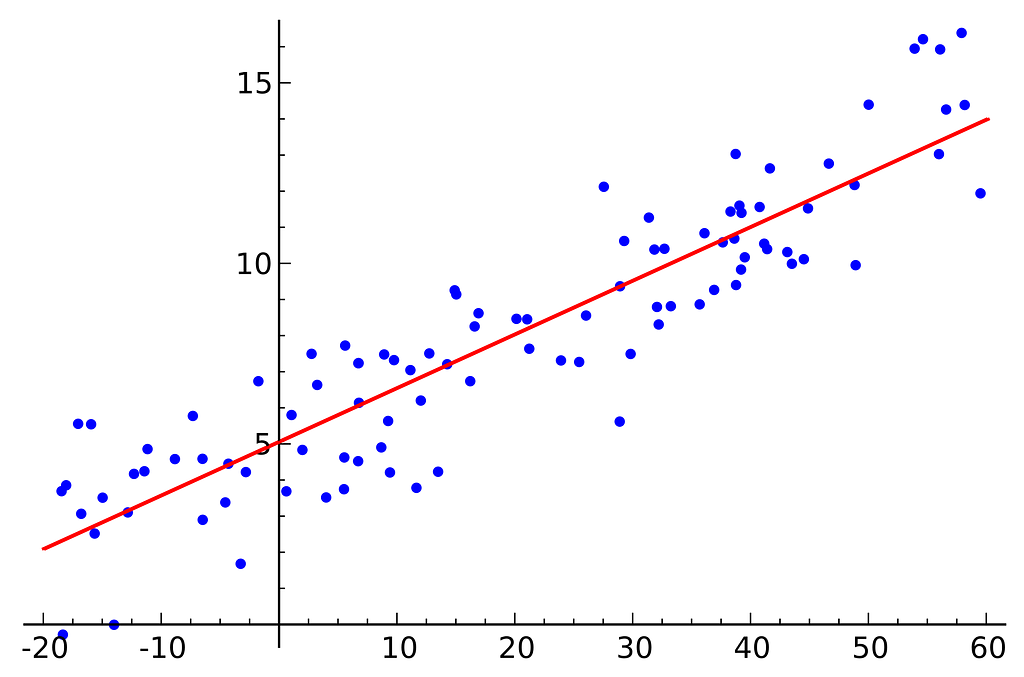Source: Wikipedia ’Linear Regression’

Since linear regression is a parametric method: where the sample data comes from a population that follows a probability distribution based on a fixed set of parameters. As such, various assumptions must be satisfied about the form of the function relating X and Y — see in the attached notes for further reading. Our model will be a function that predicts y-hat given a specific x: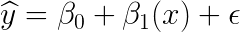This can be interpreted as, for a one unit increase in X, holding all else constant, Y increases β1

#### Interpretation

β0: is the y-intercept when x = 0. i.e. when your assignment results is 0, your predicted final exam mark is the Y intercept ( β0 )

β1: Is the slope of our line. i.e. how much does our final exam mark increase for a one unit increase in your assignment mark.

Reminder, our objective is to learn the model parameters which minimises our error term, thereby increasing the accuracy of our model’s prediction.

To find the best model parameters:
1. Define a cost function, or loss function, that measures how inaccurate our model’s prediction is.
2. Find the parameter that minimises loss, i.e. make our model as accurate as possible.

Graphically this can be represented in a Cartesian plane, as our model is two dimensional. This would change into a plane for three dimensions, etc…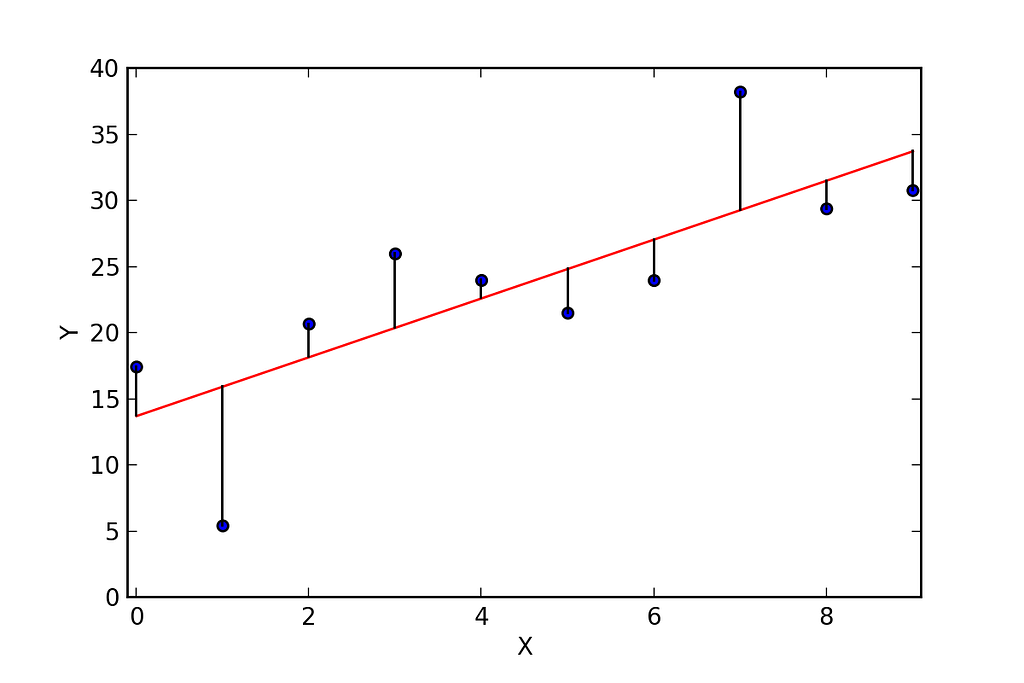Where Y is our Final Exam Mark, and X is our Assignment Mark
Note for dimensionality: our example is two-dimensional for simplicity, however, this is unrealistic and typically you will have more features (x’s) and coefficients in your model, as generally you will have more than one feature that is significant in explaining your dependent variable. In addition, linear regression suffers enormously from the curse of dimensionality, as once we deal with high-dimensional spaces, every data point becomes an outlier.

#### Cost Function

The formula for the cost function may be daunting for you at first. However, it is extremely simple and intuitive to understand.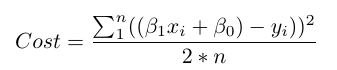Cost Function (Error Term) of our linear model

Simply, the cost function says to take the difference between each real data point (y) and our model’s prediction (ŷ), square the differences to avoid negative numbers and penalise larger differences. Finally, add them up and take the average. Except rather than dividing it by n, we divide it by 2*n. This is because mathematicians have decided that it is easier to derive. Feel free to take this to the mathematics court of justice. However, for simplicity just remember that we take 2*n.

For problems that are 2 dimensional, we can could simply derive the optimal beta parameters that minimise our loss function. However, as the model grows increasingly complex, computing the beta parameters for each variable becomes no longer feasible. As such, a method known as Gradient Descent will be necessary in allowing us to minimise our loss function.

### Gradient Descent: Learning our Parameters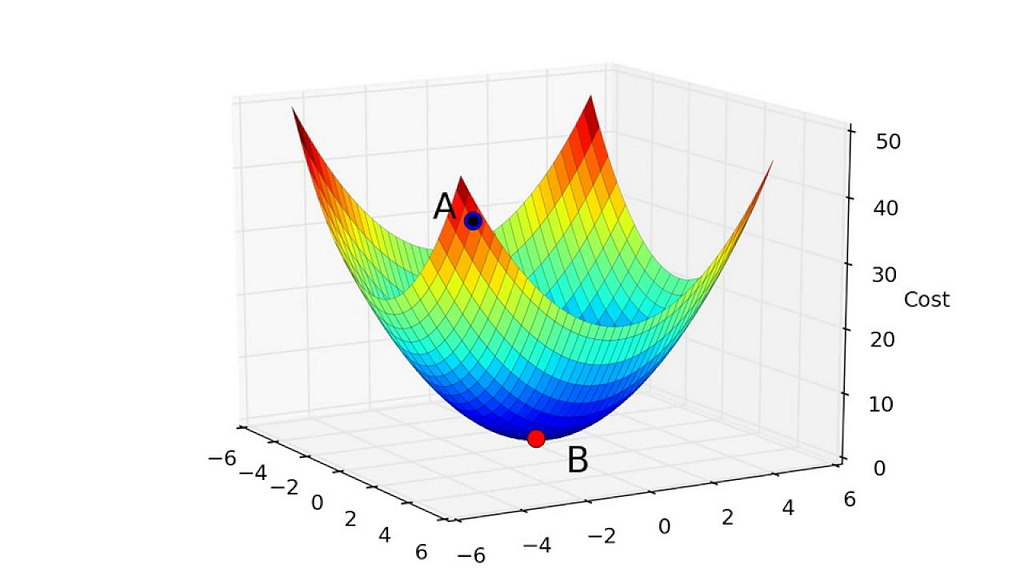Source: Youtube Mwamba Capital
Imagine you’re standing somewhere on a mountain (point A). You want to get as low as possible as fast as possible, so you decide to take the following steps:
- You check your current altitude, your altitude a step north, a step south, a step east, a step west. Using this, you figure out which direction you should step to reduce your altitude as much as possible in this step.

— Repeat until stepping any direction will cause you to go up again (point B).
This is Gradient Descent

Currently, gradient descent is one of the most popular methods used for parameter optimisation. It is often used with Neural Networks, which we will cover later. Nonetheless, it is important to understand what it does, and how it works.

The goal of gradient descent is to find the minimum point of our model’s cost function by iteratively getting a better and better approximation. This is achieved through iteratively, moving in which direction the ground is sloping down most steeply, until stepping any direction will cause you to go up again.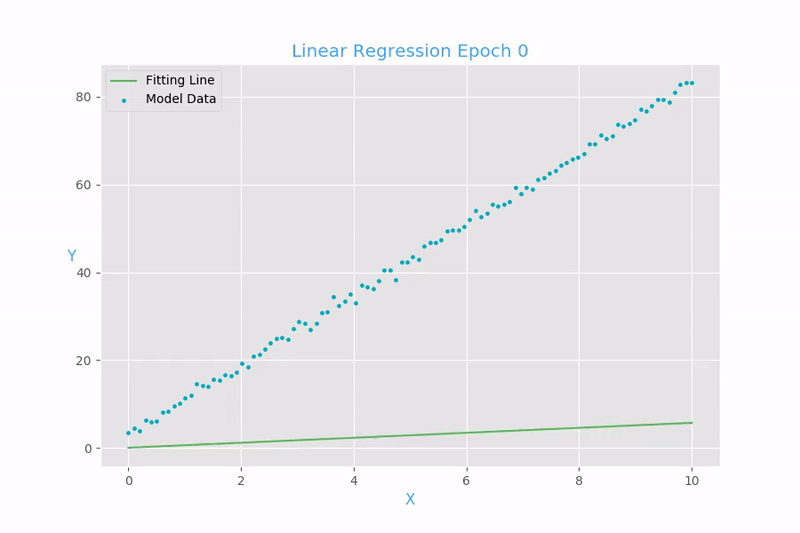Source: PyData

Taking a look at our loss function we saw in regression:

We see that this is really a function of two variables: β0 and β1. All the rest of the variables are determined, since X, Y and N are given during training. Hence, we want to try and minimise this function.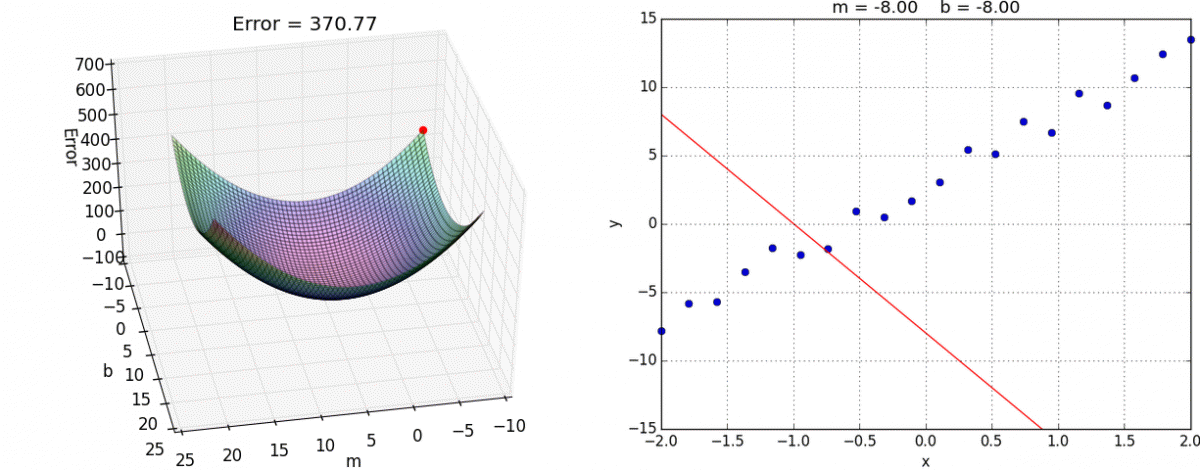Source: Github Alykhan Tejani

The function is ( β0 , β1 ) = z. To begin gradient descent, we start by making a guess of the parameters B0 and B1 that minimise the function.

Next, we take the partial derivative of the loss function with respect to each beta parameters: [dz/dβ0, dz/dβ1]. The partial derivative indicates how much total loss increased or decreased if you increase β0 or β1 by a very small amount. If the partial derivative of dz/dβ1 is a negative number, then increasing β1 is good as it will reduce our total loss. If it is a positive number, you want to decrease β1. If it is zero, we don’t change β1, as it means we have reached optimum.

We do this until we reach the bottom, i.e, the algorithm converges and the loss has been minimised.

### Overfitting

Overfitting:”Sherlock, your explanation of what just happened is too specific to the situation”. Regularisation: “Don’t overcomplicate things, Sherlock.” I’ll punch you for every extra word. Hyperparameter ( λ ):”“Here’s the strength with which I will punch you for every extra word” — Credits to Vishal Maini

Overfitting occurs when the trained model performs too well on the training data that the model learned on, but does not generalise well on the test data. The problem of overfitting is not limited to computers, humans are often no better. For instance, say you had a bad experience with an XYZ Airline, maybe the service wasn’t good, or that the airline was riddled with delays. You might be tempted to say that all flights on XYZ airline sucks. This is called overfitting whereby we overgeneralise something, which otherwise, might have been us just having a bad day.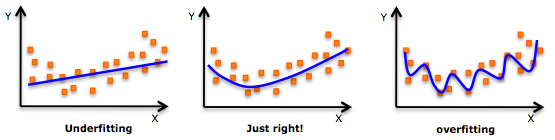Source: Quora: Luis Argerich

Overfitting occurs when a model over-learns from the training data to the point where it starts picking up idiosyncrasies that aren’t representative of patterns in the real world. This becomes especially problematic as you make your model increasingly complex. Underfitting is related to the issue where your model is not complex enough to capture the underlying trends in the data.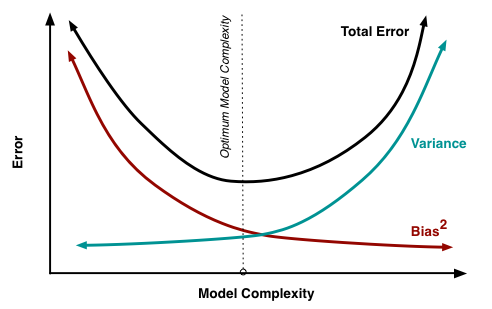Source: Scott Fortmann-Roe
``Bias-Variance Tradeoff``
``Bias: is the amount of error introduced by approximating real-world phenomena with a simplified model.``
``Variance: is how much your model’s test error changes based on variation in the training data. It reflects the model’s sensitivity to the idiosyncrasies of the data set it was trained on``
``As a model increases in complexity and it becomes flexible, its bias decreases (it does a good job of explaining the training data), but variance increases (it doesn’t generalise as well).``
Ultimately, in order to have a good model, you need one with low bias and low variance

Remember, the only thing we care about is how well the model performs on the test data. You want to predict the mark of a student’s final exam result, before they are marked, not just build a model that is 100% accurate in classifying a students mark based of the training set.

Two ways to combat overfitting:

1. Use more training data: The more you have, the harder it is to overfit the data by learning too much from any single training example.

2. User regularisation: Add in a penalty in the loss function for building a model that assigns too much explanatory power to any one feature or allows to many features to be taken into account

The first piece of the sum above is our normal cost function. The second piece is a regularisation term that adds a penalty for large beta coefficients that give too much explanatory power to any specific feature.

With these two elements in place, the cost function now balances between two priorities: explaining the training data and preventing that explanation from becoming overly specific.

The lambda coefficient of the regularisation term in the cost function is a hyperparameter: a general setting of your model that can be increased or decreased (i.e. tuned) in order to improve performance. A higher lambda value harshly penalises large beta coefficients that could lead to potential overfitting. To decide on the best value of lambda (λ), you’d use a method known as cross-validation which involves holding our a portion of the training data during training, then seeing how well your model explains the held-out portion. We’ll go over this in more depth in future series'.

``For those whom are interested in the full implementation of Supervised Learning: Linear Regression. It can be found here ``

### Wooh!! You’ve just covered the essentials of Supervised Learning: Linear Regression!

Here’s what we covered in this section:

• How supervised data can be used to enable computers to learn a function without explicitly being programmed.
• Linear Regression, the fundamentals of parametric algorithms
• Learning parameters with gradient descent
• Overfitting and regularisation

### Further Reading & Practice

For a more comprehensive understanding of linear regression, I recommend ‘Elements of Statistical Learning’ by Trevor Hastie. It’s a fantastic book which covers the essentials of Statistical Learning using Linear Algebra.

For logistic regression, I recommend ‘Applied logistic regression’ by David W. Hosmer. It goes into much more detail in the different methods and approaches available for logistic regression.

For Practice:

For practice, I recommend playing around with datasets used to predict housing prices, Boston housing data is the most popular. Otherwise, consider salary prediction.

For Datasets to considering implementing supervised learning: Linear regression. I recommend going on reddit/datasets or Kaggle, to practice and see how accurate of a prediction you can create.